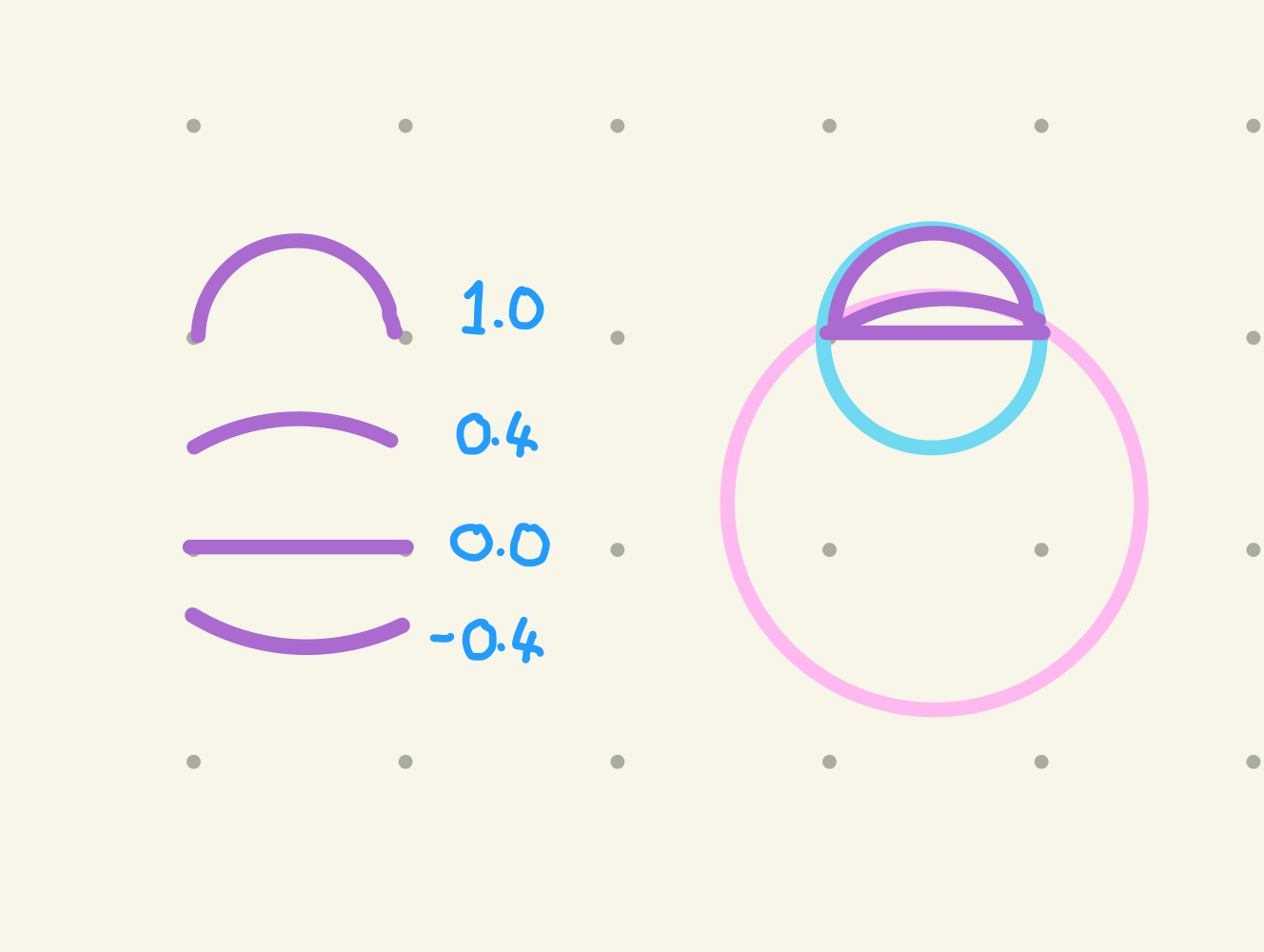# Line Curviness• updated
• DeclinedI am currently creating curved lines by using a small section of a disc, instead of a regular Line. It would be fantastic if this  could be built into the line inspector.

I'm using this technique to make flowers, but it's very difficult to setup manually, and the maths is tricky for me.

Reporting a bug? please specify Unity version:
Reporting a bug? please specify Shapes version:
Pinned repliesFreya Holmér
• Declined

I think this would be too specific of a feature to have on the line and use an extra parameter along with the specialized circular math needed in the shader, especially since it's essentially no longer a line as well as something that can be done with the arc already as is

If you want the math for it though, this might help! (requires my math library Mathfs)

`using Shapes;using UnityEngine;public class LineCurviness : MonoBehaviour {   public Vector2 start;   public Vector2 end;   [Range( -1f, 1f )] public float curviness = 0f;   void OnDrawGizmos() {      if( Mathf.Abs( curviness ) > 0.001f ) {                  // Mathfs         Vector2 normalOffset = ( end - start ).Rotate90CW() * curviness * 0.5f;         Vector2 lineCenter = ( start + end ) / 2;         Vector2 thirdPoint = lineCenter + normalOffset;         ( Vector2 center, float radius ) = Mathfs.Triangle.Circumcircle( start, end, thirdPoint );         Vector2 centerToStart = start - center;         Vector2 centerToEnd = end - center;         float angleBetween = Vector2.Angle( centerToStart, centerToEnd ) * Mathf.Deg2Rad;         float angToStart = centerToStart.Angle();         // apply         Disc disc = GetComponent<Disc>();         disc.transform.position = center;         disc.Radius = radius;         disc.AngRadiansStart = angToStart;         disc.AngRadiansEnd = angToStart + angleBetween * Mathf.Sign( curviness );      } else {         // line, not an arc      }         }}`
Replies 2Freya Holmér
• Declined

I think this would be too specific of a feature to have on the line and use an extra parameter along with the specialized circular math needed in the shader, especially since it's essentially no longer a line as well as something that can be done with the arc already as is

If you want the math for it though, this might help! (requires my math library Mathfs)

`using Shapes;using UnityEngine;public class LineCurviness : MonoBehaviour {   public Vector2 start;   public Vector2 end;   [Range( -1f, 1f )] public float curviness = 0f;   void OnDrawGizmos() {      if( Mathf.Abs( curviness ) > 0.001f ) {                  // Mathfs         Vector2 normalOffset = ( end - start ).Rotate90CW() * curviness * 0.5f;         Vector2 lineCenter = ( start + end ) / 2;         Vector2 thirdPoint = lineCenter + normalOffset;         ( Vector2 center, float radius ) = Mathfs.Triangle.Circumcircle( start, end, thirdPoint );         Vector2 centerToStart = start - center;         Vector2 centerToEnd = end - center;         float angleBetween = Vector2.Angle( centerToStart, centerToEnd ) * Mathf.Deg2Rad;         float angToStart = centerToStart.Angle();         // apply         Disc disc = GetComponent<Disc>();         disc.transform.position = center;         disc.Radius = radius;         disc.AngRadiansStart = angToStart;         disc.AngRadiansEnd = angToStart + angleBetween * Mathf.Sign( curviness );      } else {         // line, not an arc      }         }}`hayden scott-baron

I totally understand! Thanks for considering it, and wow - thanks for your script!You turned my frown upside down. :)
Thanks for making such a fantastic system!

﻿